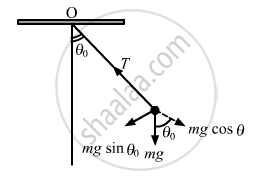Share

# Suppose the Amplitude of a Simple Pendulum Having a Bob of Mass M is θ0. Find the Tension in the String When the Bob is at Its Extreme Position. - Physics

ConceptCircular Motion

#### Question

Suppose the amplitude of a simple pendulum having a bob of mass m is θ0. Find the tension in the string when the bob is at its extreme position.

#### Solution

Let T be the tension in the string at the extreme position.
Velocity of the pendulum is zero at the extreme position.
So, there is no centripetal force on the bob.
∴ T = mgcosθIs there an error in this question or solution?

#### APPEARS IN

Solution Suppose the Amplitude of a Simple Pendulum Having a Bob of Mass M is θ0. Find the Tension in the String When the Bob is at Its Extreme Position. Concept: Circular Motion.
S